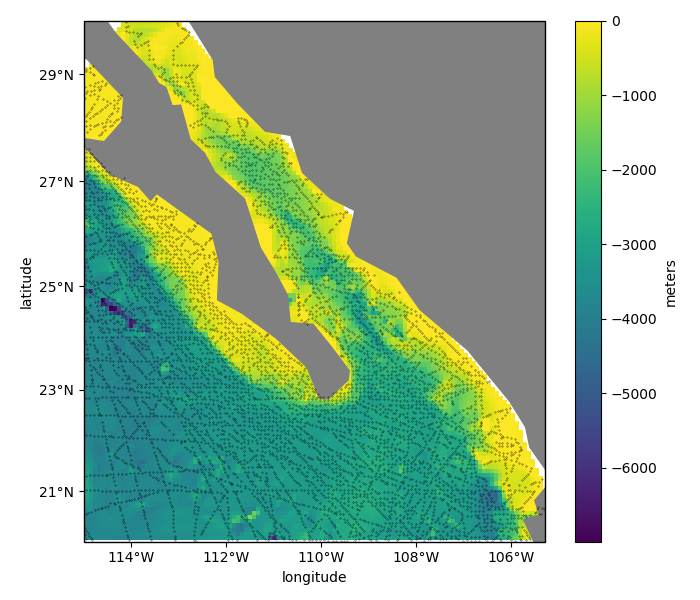# Gridding with Scipy¶

Scipy offers a range of interpolation methods in scipy.interpolate and 3 specifically for 2D data (linear, nearest neighbors, and bicubic). Verde offers an interface for these 3 scipy interpolators in verde.ScipyGridder.

All of these interpolations work on Cartesian data, so if we want to grid geographic data (like our Baja California bathymetry) we need to project them into a Cartesian system. We’ll use pyproj to calculate a Mercator projection for the data.

For convenience, Verde still allows us to make geographic grids by passing the projection argument to verde.ScipyGridder.grid and the like. When doing so, the grid will be generated using geographic coordinates which will be projected prior to interpolation.Out:

Gridder used: ScipyGridder(extra_args=None, method='cubic')
Data region: (245.0, 254.705, 20.0, 29.99131)
Generated geographic grid:
<xarray.Dataset>
Dimensions:       (latitude: 121, longitude: 117)
Coordinates:
* longitude     (longitude) float64 245.0 245.1 245.2 ... 254.5 254.6 254.7
* latitude      (latitude) float64 20.0 20.08 20.17 ... 29.82 29.91 29.99
Data variables:
bathymetry_m  (latitude, longitude) float64 nan nan nan nan ... nan nan nan
Attributes:


import matplotlib.pyplot as plt
import cartopy.crs as ccrs
import pyproj
import numpy as np
import verde as vd

# We'll test this on the Baja California shipborne bathymetry data
data = vd.datasets.fetch_baja_bathymetry()

# Before gridding, we need to decimate the data to avoid aliasing because of the
# oversampling along the ship tracks. We'll use a blocked median with 5 arc-minute
# blocks.
spacing = 5 / 60
reducer = vd.BlockReduce(reduction=np.median, spacing=spacing)
coordinates, bathymetry = reducer.filter(
(data.longitude, data.latitude), data.bathymetry_m
)

# Project the data using pyproj so that we can use it as input for the gridder.
# We'll set the latitude of true scale to the mean latitude of the data.
projection = pyproj.Proj(proj="merc", lat_ts=data.latitude.mean())
proj_coordinates = projection(*coordinates)

# Now we can set up a gridder for the decimated data
grd = vd.ScipyGridder(method="cubic").fit(proj_coordinates, bathymetry)
print("Gridder used:", grd)

# Get the grid region in geographic coordinates
region = vd.get_region((data.longitude, data.latitude))
print("Data region:", region)

# The 'grid' method can still make a geographic grid if we pass in a projection function
# that converts lon, lat into the easting, northing coordinates that we used in 'fit'.
# This can be any function that takes lon, lat and returns x, y. In our case, it'll be
# the 'projection' variable that we created above. We'll also set the names of the grid
# dimensions and the name the data variable in our grid (the default would be 'scalars',
# which isn't very informative).
grid = grd.grid(
region=region,
spacing=spacing,
projection=projection,
dims=["latitude", "longitude"],
data_names=["bathymetry_m"],
)
print("Generated geographic grid:")
print(grid)

# Cartopy requires setting the coordinate reference system (CRS) of the original data
# through the transform argument. Their docs say to use PlateCarree to represent
# geographic data.
crs = ccrs.PlateCarree()

plt.figure(figsize=(7, 6))
# Make a Mercator map of our gridded bathymetry
ax = plt.axes(projection=ccrs.Mercator())
ax.set_title("Gridded Bathymetry Using Scipy")
# Plot the gridded bathymetry
pc = grid.bathymetry_m.plot.pcolormesh(